• 自己编写的最小二乘法的小程序，用于取直线的方程，提取直线参数
• 针对电池一阶模型，使用最小二乘法进行参数辨识，使误差最小。有研究电池模型或者最小二乘法的同学可以过来看看。
• 如何用最小二乘法进行曲线的参数估计？这是日常工作中最常碰到的问题，此文一步一步为你道来！
• 最小二乘法（又称最小平方法）是一种数学优化技术。它通过最小化误差的平方和寻找数据的最佳函数匹配。利用最小二乘法可以简便地求得未知的数据，...其他一些优化问题也可通过最小化能量或最大化熵用最小二乘法来表达。
• 仅适合初学者 包含四个程序 递推最小二乘法、递推增广最小二乘法、遗忘因子递推最小二乘法、遗忘因子递推增广最小二乘法
• RLS参数辨识，最小二乘法RLS参数辨识，最小二乘法RLS参数辨识，最小二乘法
• matlab最小二乘法的非线性参数拟合首先说一下匿名函数：在创建匿名函数时，Matlab记录了关于函数的信息，当使用句柄调用该函数的时候，Matlab不再进行搜索，而是立即执行该函数，极大提高了效率。所以首选匿名函数。...
matlab最小二乘法的非线性参数拟合首先说一下匿名函数：在创建匿名函数时，Matlab记录了关于函数的信息，当使用句柄调用该函数的时候，Matlab不再进行搜索，而是立即执行该函数，极大提高了效率。所以首选匿名函数。具体拟合时可以使用的方法如下：1 曲线拟合工具箱提供了很多拟合函数，使用简单非线性拟合nlinfit函数clear all;x1=[0.4292 0.4269 0.381 0.4015 0.4117 0.3017]';x2=[0.00014 0.00059 0.0126 0.0061 0.00425 0.0443]';x=[x1 x2];y=[0.517 0.509 0.44 0.466 0.479 0.309]';f=@(p,x)2.350176*p(1)*(1-1/p(2))*(1-(1-x(:,1).^(1/p(2))).^p(2)).^2.*(x(:,1).^ (-1/p(2))-1).^(-p(2)).*x(:,1).^(-1/p(2)-0.5).*x(:,2);p0=[8 0.5]';opt=optimset('TolFun',1e-3,'TolX',1e-3);%[p R]=nlinfit(x,y,f,p0,opt)2 最小二乘法在曲线拟合中比较普遍。拟合的模型主要有1.直线型2.多项式型3.分数函数型4.指数函数型5.对数线性型6.高斯函数型一般对于LS问题，通常利用反斜杠运算“\”、fminsearch或优化工具箱提供的极小化函数求解。在Matlab中，曲线拟合工具箱也提供了曲线拟合的图形界面操作。在命令提示符后键入：cftool，即可根据数据，选择适当的拟合模型。“\”命令1.假设要拟合的多项式是：y=a+b*x+c*x^2.首先建立设计矩阵X：X=[ones(size(x)) x x^2];执行：para=X\ypara中包含了三个参数：para(1)=a;para(2)=b;para(3)=c;这种方法对于系数是线性的模型也适应。2.假设要拟合：y=a+b*exp(x)+cx*exp(x^2)设计矩阵X为X=[ones(size(x)) exp(x) x.*exp(x.^2)];para=X\y3.多重回归(乘积回归)设要拟合：y=a+b*x+c*t，其中x和t是预测变量，y是响应变量。设计矩阵为X=[ones(size(x)) x t] %注意x,t大小相等！para=X\y
展开全文• 参数提取代码以最小二乘法将已知模型拟合到给定的测量数据。 它适用于任意数量的变量和参数。 所使用的数值微分方法是具有简单线搜索的简单拟牛顿法。 这种方法适用于简单的问题，但随着问题变得更大和嘈杂，对...matlab
• 基于MIMO系统递推最小二乘法参数辨识程序，能
• 文章对经典的最小二乘和全最小二乘方法的应用背景 原理与算法进行了介绍 给出了它们在线性模型参数估计中的 实现 通过计算机仿真说明了在模型中所有变量均具有不可忽略的误差时 全最小二乘法得到的参数估计更接近于...
• 给定点数N，和N个点的坐标 利用最小二乘法计算出此N个点的拟合直线y=kx+b中的参数k和b vc6 控制台 调试通过 输入的N和坐标可自行在代码中修改 小弟初学，希望与大家交流
• 本程序为应用Matlab编写，基于递推最小二乘法进行系统参数识别的仿真。
• ## 最小二乘法

万次阅读 多人点赞 2018-01-07 15:20:04
回归学习最常用的损失函数是平方损失函数，在此情况下，回归问题可以著名的最小二乘法来解决。最小二乘法就是曲线拟合的一种解决方法。 最小二乘法的问题分为两类： 线性最小二乘法 非线性最小二乘法 如果是...
目录最小二乘法的思想目标公式求解方法矩阵求导方法最小二乘和梯度下降区别简单总结后记线性回归python参考
最小二乘法的思想
最小二乘法则是一种统计学习优化技术，它的目标是最小化误差平方之和来作为目标，从而找到最优模型，这个模型可以拟合（fit）观察数据。
回归学习最常用的损失函数是平方损失函数，在此情况下，回归问题可以用著名的最小二乘法来解决。最小二乘法就是曲线拟合的一种解决方法。
最小二乘法的问题分为两类：
线性最小二乘法
非线性最小二乘法
如果是线性的则有闭式解(closed-form solution)，唯一解。理解为所有点都在某条线上，全拟合好了。
非线性的经常需要数值方法来求解。比如：随机梯度下降或者牛顿法等。当然，随机梯度下降也可以解决线性问题。
目标公式
J(θ)=∑i=1m(fθ(xi)−yi)2(1)J(\theta)= \sum_{i=1}^{m} (f_\theta(x_{i})-y_{i})^2 \tag{1}
最小二乘法的目标就是最小化公式1。f则是模型（取自假设空间），y则是观察值。
通俗来讲，就是观察值和拟合值(模型给出）之间的距离平方和最小化作为目标来优化。
求解方法
矩阵求导方法
思想就是把目标函数划归为矩阵运算问题，然后求导后等于0，从而得到极值。以线性回归问题为例：
求解最小二乘的问题推导为如下：求解变量θ\theta,满足(XTX)θ=XTy(2)
(X^T X)\theta = X^Ty {\tag2}

如果可逆，将得到：θ=(XTX)−1XTy
\theta = (X^T X)^{-1}X^Ty

这是利用矩阵得到的最小二乘法的一种解法。
注意这是线性回归的最小二乘法的求解结果，不是其他问题的，其他问题的假设函数有时候很复杂。比如下面的博文对线性回归的推算挺好，但没有说明求导的大前提条件：线性回归，这容易把最小二乘法和最小二乘法的求解混在一起。
http://blog.csdn.net/ACdreamers/article/details/44662633
##数值方法随机梯度下降
思路：对参数向量求导，使其梯度为0，然后得到参数变量的迭代更新公式。θj:=θj−α∗∂J(θ)∂(θj)(3)\theta_j:=\theta_j - \alpha* \frac{\partial J(\theta)}{\partial(\theta_j)} \tag{3}
请参考：http://blog.csdn.net/iterate7/article/details/76709492
##数值方法牛顿法
利用泰勒公式展开，利用梯度和海塞矩阵进行迭代下降。速度很快。xk+1=xk−Hk−1gk(4)x^{k+1}=x^{k}-H^{-1}_kg_{k}
\tag{4}
变量以牛顿方法来下降。
牛顿方向定义为：−Hk−1gk
-H^{-1}_kg_{k}

请参考：
http://blog.csdn.net/iterate7/article/details/78387326
最小二乘和梯度下降区别
最小二乘看做是优化问题的话，那么梯度下降是求解方法的一种。梯度下降是一种解决最优化问题的数值方法。最小二乘法则是一个最优化问题。
简单总结
数值方法的基本含义则是对一个函数求极值，在无法直接求得解析解的情况下，通过求导为0的方法，找到迭代方向保证可以下降目标值，梯度方向或者牛顿方向等等。 逐步下降直到满足一些工程要求则结束迭代。
后记
最小二乘法是一种对于偏差程度的评估准则思想，由公式1给出。个人认为，应该称之为：最小二乘准则。
公式1里没有给出f的值，也就是说假设空间。如果是线性回归，也就是wx+b的形式，那么公式2就是最小二乘法的解。所以大部分的博文都在此范畴讨论。为何都用这个线性回归直接说呢，其实最小二乘准则更适合线性回归。应该称之为狭义的最小二乘方法，是线性假设下的一种有闭式解的参数求解方法，最终结果为全局最优。
梯度下降只是数值求解的具体操作，和最小二乘准则下面的最优化问题都可以用随机梯度下降求解。
线性回归python
import numpy as np
import matplotlib.pyplot as plt
data = np.array([
[1, 6],
[2, 5],
[3, 7],
[4, 10]
])
m = len(data)
X = np.array([np.ones(m), data[:, 0]]).T
print("X:", X)
y = np.array(data[:, 1]).reshape(-1, 1)
print("y:",y)
W = np.linalg.solve(X.T.dot(X), X.T.dot(y)) ## 求解XW=y的值，W
print("W:",W)

##show
plt.figure(1)
xx = np.linspace(0, 5, 2)
yy = np.array(W + W * xx)
plt.plot(xx, yy.T, color='b')
plt.scatter(data[:, 0], data[:, 1], color='r')
plt.show()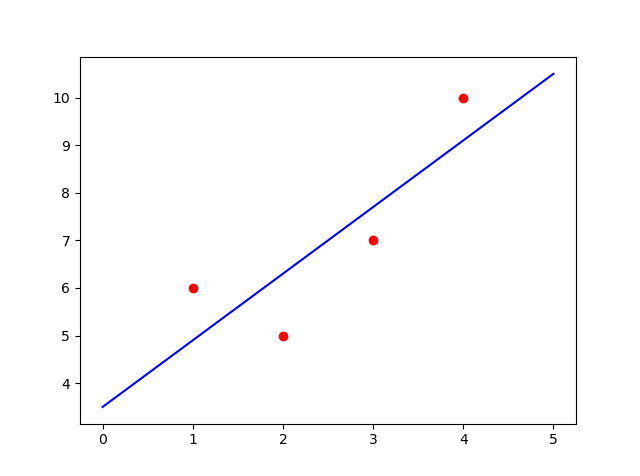参考
机器学习 周志华 线性模型和最小二乘法
https://en.wikipedia.org/wiki/Least_squares
https://en.wikipedia.org/wiki/Linear_least_squares_(mathematics)
https://en.wikipedia.org/wiki/Newton’s_method_in_optimization


展开全文随机梯度下降 梯度 最小二乘准则
• 一步完成最小二乘法、递推最小二乘法、增广最小二乘法、广义最小二乘法、辅助变量法、二步法辨识如下模型的参数： 噪声的成形滤波器 采样时间0.01 要求： 1.matlab 写出程序代码； 2.画出实际模型和...
用一步完成最小二乘法、递推最小二乘法、增广最小二乘法、广义最小二乘法、辅助变量法、二步法辨识如下模型的参数：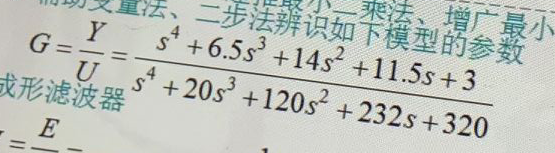噪声的成形滤波器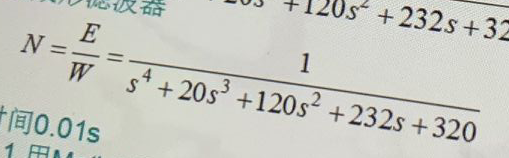采样时间0.01

要求：

1.用matlab 写出程序代码；

2.画出实际模型和辨识得到的模型误差；

3.画出递推算法迭代时各辨识参数的变化曲线；

具体思路：

采用Z变换将系统和噪声滤波器的传递函数进行离散化，得到系统的差分方程：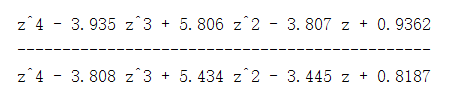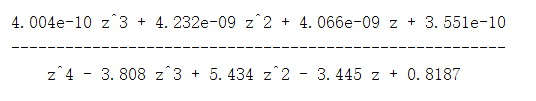产生m序列，获得系统输入：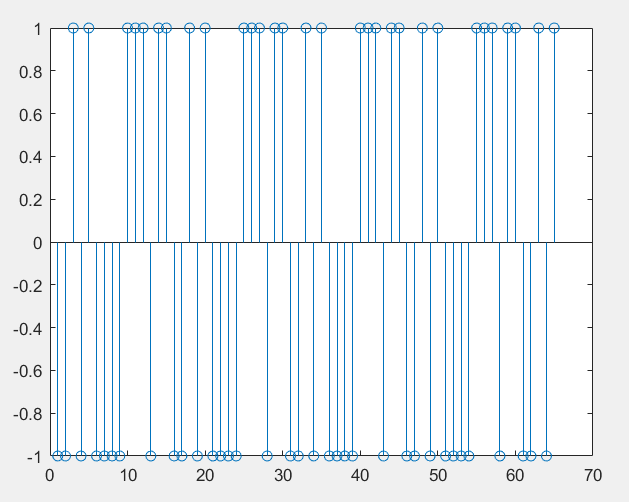然后利用各最小二乘法的公式，进行迭代求解：

增广最小二乘（辨识结果）

-0.0865   -0.1558    0.0116   -0.0125   -0.0031   -0.0198    0.0418    0.0061    0.0530    0.0152   -0.0220    0.0037    0.0894   -0.0027   -0.4287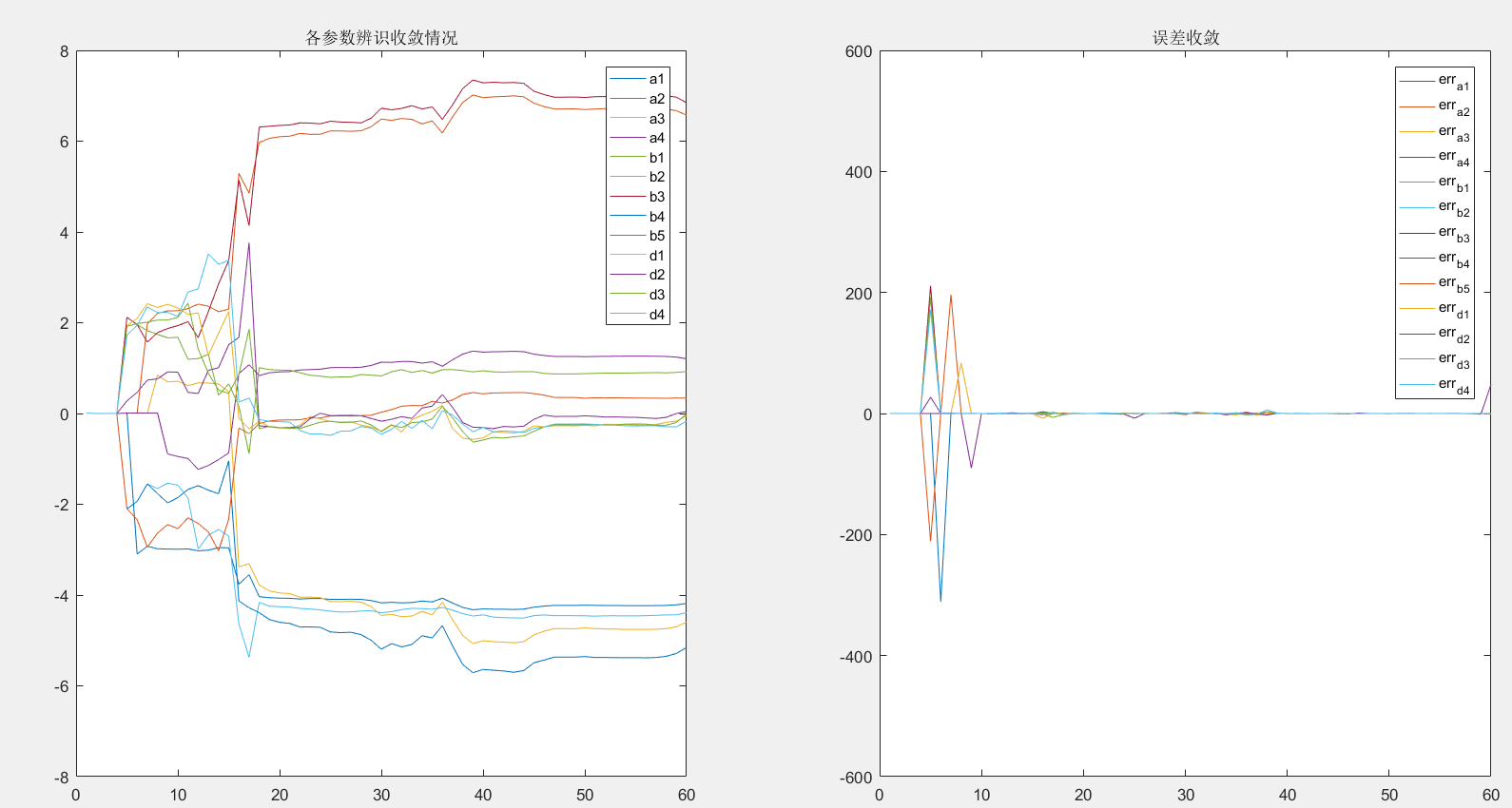递推最小二乘辨（辨识结果）

0.2914    0.4161   -0.0060   -0.0947   -0.1967    0.0093    0.0756   -0.2644    0.0262   -0.2307   -0.0284   -0.0255   -0.0943    0.0701   -0.3491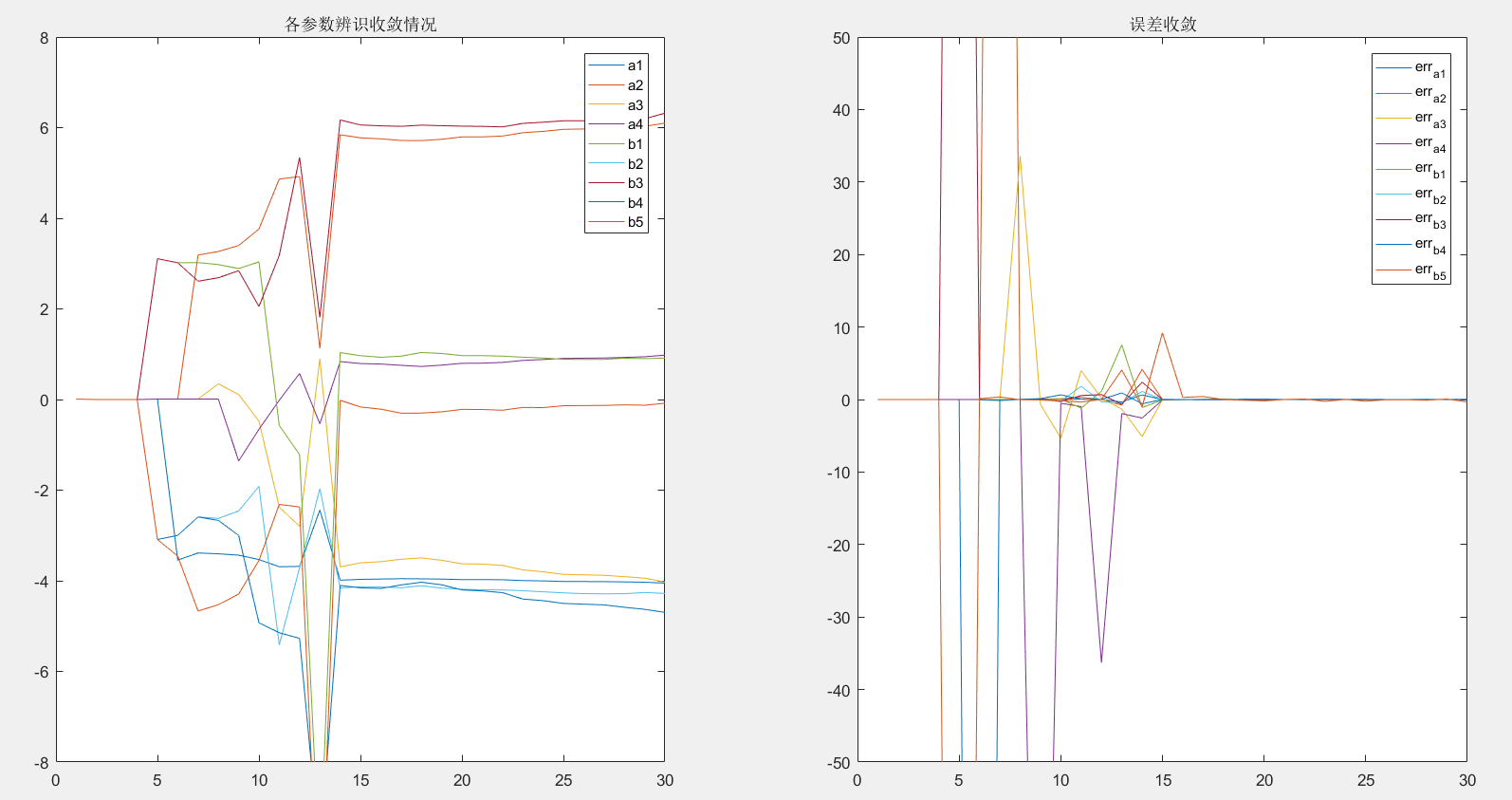二步法（辨识结果）

10.9249  -11.5145    4.9972   -4.1236   10.4250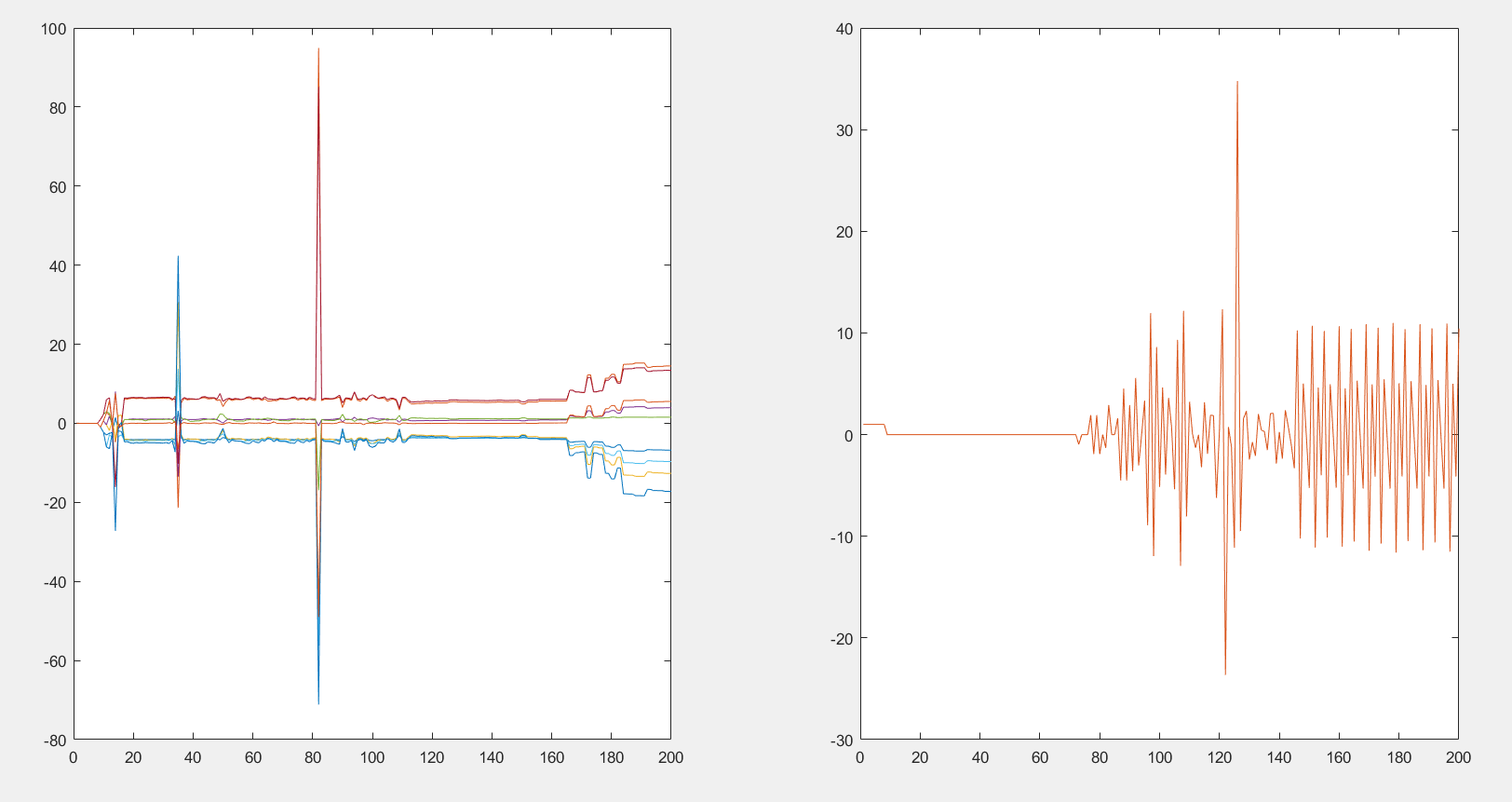最小二乘法（辨识结果）

1.5000    0.7000    1.0000    0.5000

辅助变量法（辨识结果）

0   -2.2276   -0.0000    3.4309   -2.0860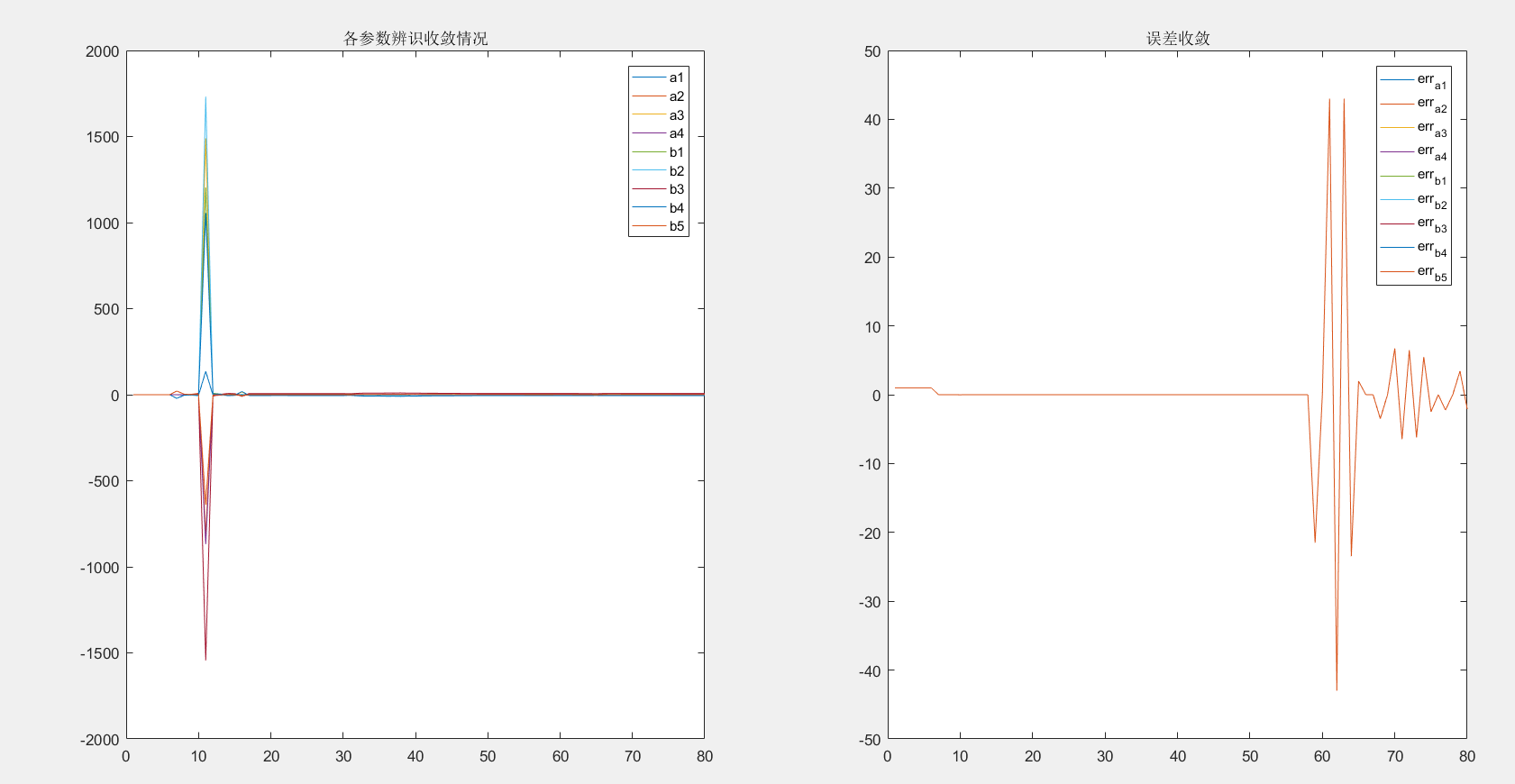展开全文系统辨识
• 递推最小二乘法：当矩阵维数增加时，矩阵逆运算计算量过大，而且不适合在线辨识。为了减少计算量，并且可以实时地辨识出动态系统的特性，可以将最小二乘法转换成参数递推的估计。 取前N组数据，然后从N+1项后向后...
一、递归最小二乘法
递推最小二乘法：当矩阵维数增加时，矩阵求逆运算计算量过大，而且不适合在线辨识。为了减少计算量，并且可以实时地辨识出动态系统的特性，可以将最小二乘法转换成参数递推的估计。
取前N组数据，然后从N+1项后向后迭代，当前估计值=上一次估计值+修正值。
给定过程：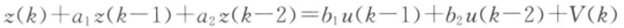式中，理想的系数值a1=1.5,a2=0.7,b1=1.0和b2=0.5。V(k)服从N(0,1)的随机噪声。
clear all
close all
clc
%产生N(0,1)正态分布的随机噪声
randn('seed',100);
v=randn(1,60);

%产生M序列
L=60;% M序列的周期
y1=1;y2=1;y3=1;y4=0;%四个移位积存器的输出初始值
for i=1:L;
x1=xor(y3,y4);
x2=y1;
x3=y2;
x4=y3;
y(i)=y4;
if y(i)>0.5,u(i)=-5;%M序列的幅值为5
else u(i)=5;
end
y1=x1;y2=x2;y3=x3;y4=x4;
end
figure(1);
stem(u),grid on
% 递推最小二乘辨识程序
z(2)=0;z(1)=0;
%观测值由理想输出值加噪声
for k=3:60;%循环变量从3到15
z(k)=-1.5*z(k-1)-0.7*z(k-2)+u(k-1)+0.5*u(k-2)+0.5*v(k);
end
%RLS递推最小二乘辨识
c0=[0.001 0.001 0.001 0.001]';
p0=10^3*eye(4,4);
E=0.000000005;%相对误差
c=[c0,zeros(4,59)];%被辨识参数矩阵的初始值及大小
e=zeros(4,60);%相对误差的初始值及大小
lamt=1;
for k=3:60;
h1=[-z(k-1),-z(k-2),u(k-1),u(k-2)]';
k1=p0*h1*inv(h1'*p0*h1+1*lamt);%求出K的值
new=z(k)-h1'*c0;
c1=c0+k1*new;%求被辨识参数c
p1=1/lamt*(eye(4)-k1*h1')*p0;
e1=(c1-c0)./c0;%求参数当前值与上一次的值的差值
e(:,k)=e1; %把当前相对变化的列向量加入误差矩阵的最后一列
c(:,k)=c1;%把辨识参数c 列向量加入辨识参数矩阵的最后一列
c0=c1;%新获得的参数作为下一次递推的旧参数
p0=p1;
if norm(e1)<=E
break;%若参数收敛满足要求，终止计算
end
end
%分离参数
a1=c(1,:); a2=c(2,:); b1=c(3,:); b2=c(4,:);
ea1=e(1,:); ea2=e(2,:); eb1=e(3,:); eb2=e(4,:);
figure(2);
i=1:60;
plot(i,a1,'k',i,a2,'b',i,b1,'r',i,b2,'g') %画出辨识结果
legend('a1','a2','b1','b2');
title('递推最小二乘参数辨识')
figure(3);
i=1:60;
plot(i,ea1,'k',i,ea2,'b',i,eb1,'r',i,eb2,'g') %画出辨识结果的收敛情况
legend('a1','a2','b1','b2');
title('辨识精度')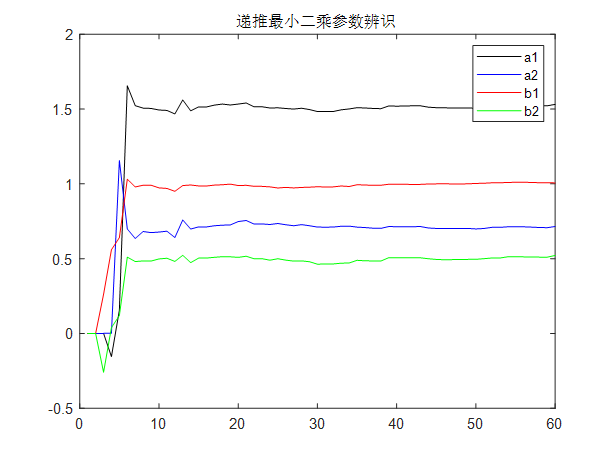二、增广最小二乘法
增广最小二乘法：当噪声均值为0时，最小二乘法参数估计算法为无偏估计，为了解决最小二乘法参数估计的有偏性，将噪声模型的辨识同时考虑进去，就是增广最小二乘法。
增广最小二乘法相当于参数向量和数据向量维数扩大了的最小二乘法，它能在有色噪声(可用平均滑动模型来表示)情况下给出参数的一致估计量，同时可以把噪声模型也辨识出来。
给定过程：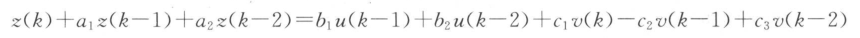式中，理想的系数值a1=1.5,a2=0.7,b1=1.0和b2=0.5,c1=1.2,c2=-1.0,c3=0.2。V(k)服从正态分布的白噪声N(0,1)，输入信号采用4阶M序列，其幅值为1。
clear all
close all
clc

% M序列、噪声信号产生及其显示程序
L=60;%四位移位积存器产生的M序列的周期
y1=1;y2=1;y3=1;y4=0;
for i=1:L;
x1=xor(y3,y4);
x2=y1;
x3=y2;
x4=y3;
y(i)=y4;
if y(i)>0.5,u(i)=-1;
else u(i)=1;
end
y1=x1;y2=x2;y3=x3;y4=x4;
end
figure(1);
stem(u),grid on%画出M序列输入信号
randn('seed',100)
v=randn(1,60); %产生一个N（0,1）的随机噪声
%增广递推最小二乘辨识
z(2)=0;z(1)=0;
theat0=[0.001 0.001 0.001 0.001 0.001 0.001 0.001]';%直接给出被辨识参数的初始值,即一个充分小的实向量
p0=10^4*eye(7,7);%初始状态P0
theat=[theat0,zeros(7,59)];%被辨识参数矩阵的初始值及大小
for k=3:60;
z(k)=-1.5*z(k-1)-0.7*z(k-2)+u(k-1)+0.5*u(k-2)+1.2*v(k)-v(k-1)+0.2*v(k-2)
h1=[-z(k-1),-z(k-2),u(k-1),u(k-2),v(k),v(k-1),v(k-2)]';
x=h1'*p0*h1+1;
x1=inv(x);
k1=p0*h1*x1; %K
d1=z(k)-h1'*theat0;
theat1=theat0+k1*d1;%辨识参数c
theat0=theat1;%给下一次用
theat(:,k)=theat1;%把辨识参数c 列向量加入辨识参数矩阵
p1=p0-k1*k1'*[h1'*p0*h1+1];%find p(k)
p0=p1;%给下次用
end%循环结束

%分离变量
a1=theat(1,:); a2=theat(2,:); b1=theat(3,:); b2=theat(4,:);
c1=theat(5,:); c2=theat(6,:); c3=theat(7,:);
i=1:60;
figure(2);
plot(i,z)
figure(3)
plot(i,a1,'r',i,a2,'b',i,b1,'k',i,b2,'y',i,c1,'g',i,c2,'c',i,c3,'m')%画出各个被辨识参数
title('增广递推最小二乘辨识方法')%标题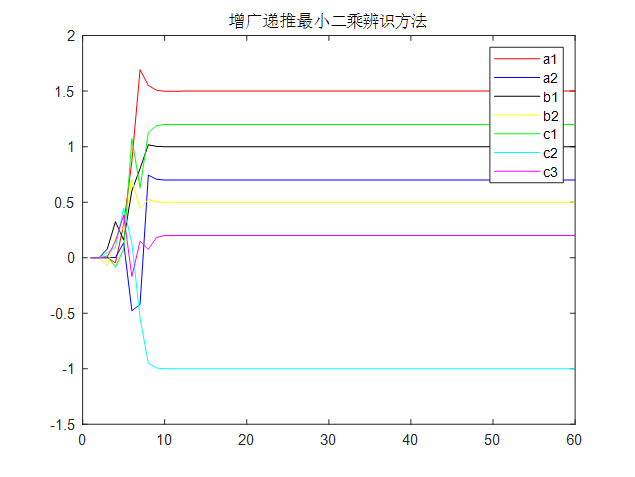三、带遗忘因子的递归增广最小二乘法的参数估计
遗忘因子算法的基本思想为：对老数据乘以遗忘因子，以降低老数据所提供的信息量，增加新数据的信息量。前一时刻的数据乘以较小的权，后一时刻的数据乘以较大的权，表示对新的数据较为重视，对老数据所起的作用逐渐削弱。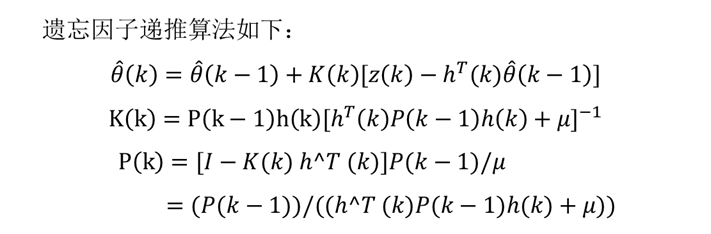%开环系统参数辨识，带遗忘因子的递推最小二乘估计法（FFRLS)，系统为单入单出的CAR(带控制量的自回归模型）模型,三阶系统
clear all
clc
a=[1 -1.1 0.6 0.1];b=[1 0.7];d=4; %实际模型系数矩阵与纯迟延
L=1000;                           %仿真长度
na=length(a)-1;nb=length(b)-1;    %na,nb为输出输入系数矩阵A,B的阶数
yk=zeros(na,1);                   %输出矩阵初始化
yk_m=zeros(na,1);                 %模型输出
uk=zeros(nb+d,1);                 %输入矩阵初始化
theta_e0=zeros(na+nb+1,1);        %theta_e0为估计参数初值，a1,a2....an,b0,b1,...bn,共na+nb+1个
phi=zeros(na+nb+1,1);             %phi为当前实际输出输入构成的矩阵
P=10^6*eye(na+nb+1);              %修正系数初值
beta=0.99;                        %遗忘因子，在0.95到1之间
u=randn(L,1);                     %输入信号，方差为1的白噪声序列
omega=sqrt(0.1)*randn(L,1);       %干扰信号，方差为0.1的白噪声序列

for i=1:L
theta(:,i)=[a(2:na+1),b]';    %系统实际参数值

phi=[-yk;uk(d:d+nb)];         %系统输出输入矩阵
phi_e=[-yk_m;uk(d:d+nb)];     %模型输出输入矩阵
y(i)=phi'*theta(:,i)+omega(i);%系统实际输出
y_m(i)=phi_e'*theta_e0;       %模型输出

%递推公式
K=P*phi/(beta+phi'*P*phi);
theta_e(:,i)=theta_e0+K*(y(i)-phi'*theta_e0);
P=(eye(na+nb+1)-K*phi')*P/beta;

%数据更新
theta_e0=theta_e(:,i);
for j=na:-1:2
yk(j)=yk(j-1);
yk_m(j)=yk_m(j-1);
end
yk(1)=y(i);
yk_m(1)=y_m(i);

for j=(nb+d):-1:2
uk(j)=uk(j-1);
end
uk(1)=u(i);
end

figure
x=[1:L];
plot(x,theta_e,x,theta,'k:');
legend('a1','a2','a3','b1','b2');
axis([0 L -2 2]);
figure
plot(x,y,x,y_m,'r');
legend('实际输出','模型输出');
axis([0 L -20 20]);
grid on;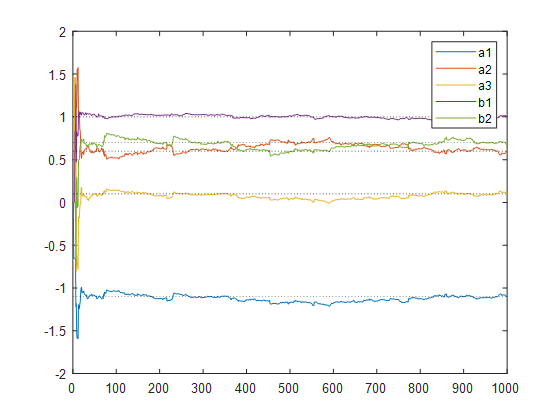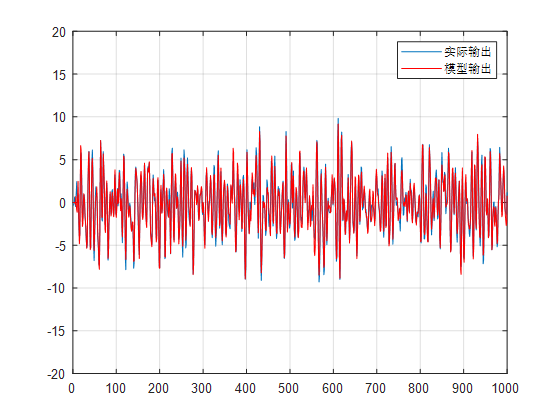参考文献：

方桂花,王鹤川,高旭.基于动态遗忘因子递推最小二乘法的永磁同步电机参数辨识算法[J].计算机应用与软件,2021,38(01):280-283.
冯亦奇,陈勇.基于遗忘因子的UKF车辆状态参数估计算法[J].合肥工业大学学报(自然科学版),2020,43(11):1450-1455+1499.
焦慧方,陈希亮,高敏,马忠玲.基于带遗忘因子交替广义最小二乘法的多变量耦合系统参数辨识[J].咸阳师范学院学报,2018,33(02):49-53.


展开全文系统辨识 遗忘因子
• 最小二乘法最小二乘法（又称最小平方法）是一种数学优化技术。它通过最小化误差的平方和寻找数据的最佳函数匹配。利用最小二乘法可以简便地求得未知的数据，并使得这些求得的数据与实际数据之间误差的平方和为最...
• # par为拟合参数的初始值 # args为需要拟合的实验数据 def get_related_par(x, y, par, residuals):  plsq = leastsq(residuals, par, args=(y, x)) # 调用leastsq进行数据拟合, residuals为计算误差的函数  ...leastsq
• 使用最小二乘法对采样后的信号进行参数估计。可以有效识别原始信号基波以及各次谐波的幅值和相位。文件包括供Matlab使用的两个m文件，以及参考的两篇文献，帮助大家更深入地理解。
• 最小二乘法用于参数估计，用于检测和参数估计.c语言实现。六个方程估计3个参数
• 基于遗忘因子的最小二乘法电池参数辨识   最小二乘法是系统辨识中最常用的一种估算方法。为了克服最小二乘法存在”数据饱和”的问题，我们通常采用含有遗忘因子的递推最小二乘法(Forgetting Factor Recursive ...
• 1.RLS递推最小二乘法原理 2.PMSM-RLS参数辨识模型 3.辨识结果simulink
• 最小二乘法非线性曲线参数拟合-最小二乘法原理及其MATLAB实现.pdf （初学者--我）最近正在研究matlab最小二乘法非线性拟合问题，昨天在论坛里求教，但没有人给予解答。只好自己查找相关文献，觉得其中最有用的一篇...matlab
• 介绍最小二乘法参数估计，计算系统参数模型的原理，以及推导过程
• 最小二乘参数辨识，是同济大学汽车学院的系统辨识课件，126页，看着不错，希望大家分享
• 最小二乘法求回归直线方程的推导过程 2017-02-07 16:38:50Neo-T阅读数 91965更多 分类专栏：数学 版权声明：本文为博主原创文章，遵循CC 4.0 BY-SA版权协议，转载请附上原文出处链接和本声明。 本文链接：...
• 此示例使用非线性最小二乘法解决涉及参数池化和分层的复杂曲线拟合问题。 此示例还利用了 MATLAB 7 的一些新语言功能。 * 匿名函数*嵌套函数matlab
• 基于最小二乘法的系统参数辨识，吴令红，熊晓燕，系统辨识是自动控制学科的一个重要分支，由于其特殊作用，已经广泛应用于各种领域，尤其是复杂系统或参数不容易确定的系统的建模机器学习 算法 多元线性回归...